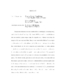## Plasma-neutral equilibrium in centrifugally confined plasma##### Files
umi-umd-4510.pdf(1.47 MB)
Plasma-neutral interactions are considered for a centrifugally confined plasma, such as the Maryland Centrifugal eXperiment (MCX), wherein a crossfield plasma rotation inhibits plasma escape along the magnetic field. Interactions along the magnetic field are considered first. Analytic and numerical solutions from a simple one-dimensional isothermal model are obtained. It is shown that for perfect recycling the neutral density at the wall is exponentially smaller than the central plasma density for strong centrifugal confinement compared to the case of no confinement for which the neutral wall density equals the central plasma density. The exponential factor is effectively $\exp\left(-M_s^2/2\right)$, where $M_s$ is the sonic Mach number of the rotation speed. The effective neutral penetration depth along the field, of the same order as the crossfield penetration depth in the zero confinement limit, increases significantly in the strong confinement case. From the one-dimensional cold-ion calculation, the ratio of the neutral densities at the end-wall to the side-wall, $N_{||}/N_\bot$, is much larger than unity for weak confinement. But when $M_s$ is about $3$, the two densities are about equal and the inequality reverses beyond that. We next extend an existing MHD numerical code to include the neutral fluid, allowing two dimensional study. Slab geometry has been used with a reasonable force model for the confinement mechanism. We found that when the rotation Mach number is about $3.7$, $N_{||}/N_\bot=1$. The experimental relevant interaction parameter, $nN$, is also shown to be peaking at the side-wall rather than the end-wall when confinement is strong. A preliminary study in MCX geometry is also carried out giving first results for more realistic 2D structures. Finally, an analytical study of momentum confinement time due to dissipative processes at the insulator is commenced. The momentum loss along the field line to the insulating end-wall might be a concern. Classical Hartmann theory suggests that the MCX results would not be obtainable. By including the Hall effect in the Hartmann problem, an analytical solution is found and has the potential to increase the momentum confinement time by factor of $\sqrt{\epsilon/\eta}$ where $\epsilon=c/\omega_{pi}L$ and $\eta$ is resistivity normalized to $4\pi LVa/c^2$.